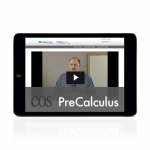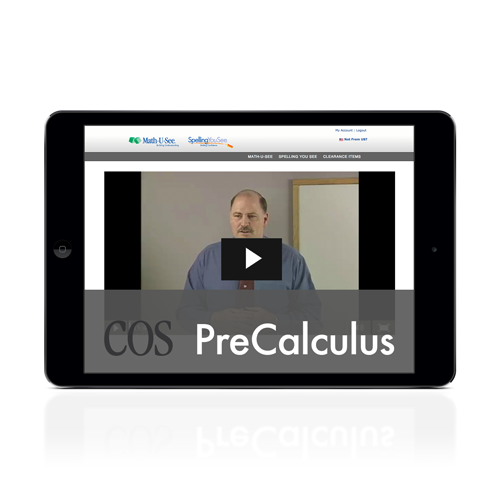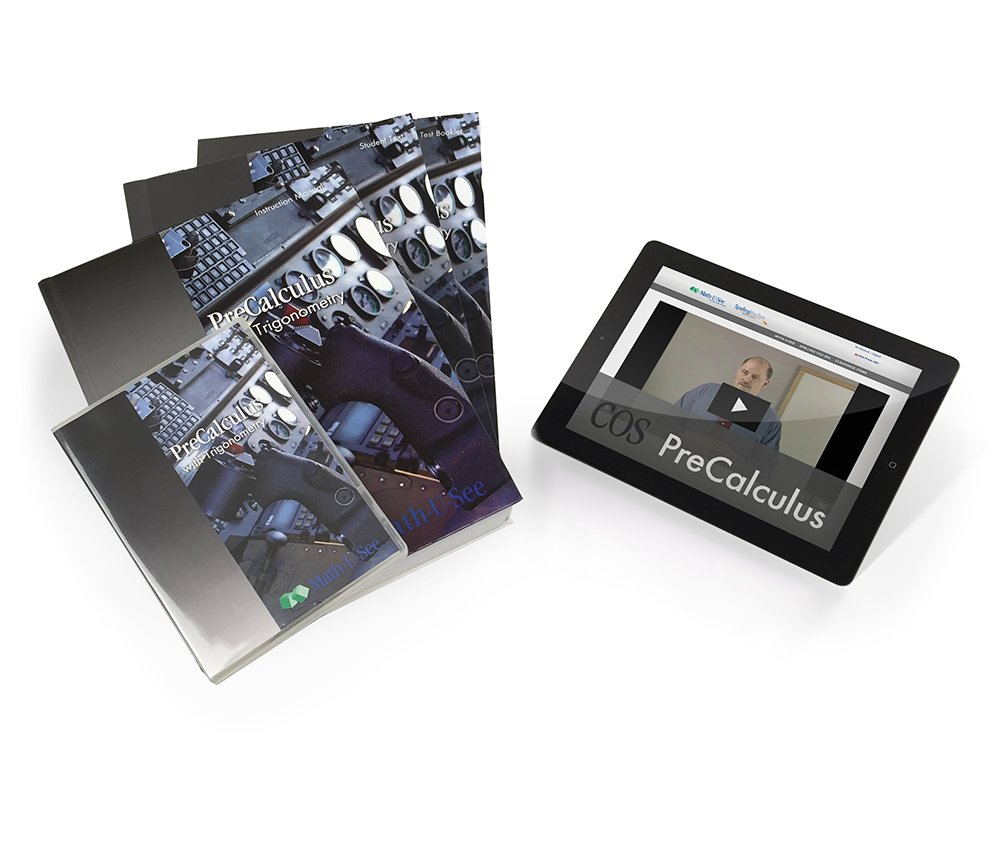## PreCalculus ProductsIncludes everything you need for a successful experience with PreCalculus:

• Instruction Manual with complete solutions
• Instruction DVD
• Student Workbook
• Tests booklet

Order HereThe PreCalculus Student Pack contains the Student Workbook with lesson-by-lesson worksheets and review pages and the PreCalculus Tests.

Order HereThe PreCalculus Instruction Pack contains the instruction manual with lesson-by-lesson instructions and detailed solutions, and the DVD with lesson-by-lesson video instruction..

Order HereLifetime access to PreCalculus streaming instruction videos and online Instruction Manual, lesson and test solutions, and other online resources from any browser. You will be sent Access instructions once your pack has been set up. Note: Digital Packs do not include Student Workbook or Tests..

Order Here

# PreCalculus

### Trigonometry, identities, polar equations, logarithms, sequences, limits and other topics to prepare for calculus.

Major Concepts and Skills Include:

• Understanding and working with trigonometric ratios, their reciprocals, and their inverses
• Working with trigonometric expressions and identities
• Understanding and working with polar coordinates and radian measure
• Graphing and analyzing trigonometric functions
• Understanding functions, limits, domain, and range
• Working with Arithmetic and Geometric Series and Sequences

Additional Concepts and Skills:

• Understanding and applying the Laws of Sines and Cosines
• Understanding and applying the Sum, Difference, Double-Angle, and Half-Angle Identities
• Proving trigonometric identities
• Working with vectors and adding linear functions
• Understanding and working with logarithms
• Working with Euler’s number and natural logarithms

## View PreCalculus Sample Lesson PDF

• Pythagorean Theorem
• Special Right Triangles (30°- 60°- 90° and 45°- 45°- 90°)
• Trigonometric Ratios
• Inverse Trigonometric Ratios
• Interpreting the Trigonometry Tables
• Using the Trig. Table to Solve for the Unknown
• Using a Calculator and Arc Functions
• Angles of Elevation and Depression
• Angles less than 0°
• Angles greater than 360°
• Reference Angles
• Cofunctions
• Negative Angle Relationships
• Proving Trigonometric Identities
• Sum and Difference Identities
• Double-Angle and Half-Angle Identities
• Law of Sines
• Law of Cosines
• Ambiguity in the Law of Sines
• Polar Coordinates and Rectangular Coordinates
• Polar Equations and Polar Graphs
• Vectors
• Functions: Relation, Domain, and Range
• Graphing the Sine and Cosine Functions
• Graphing the Secant and Cosecant Functions
• Graphing the Tangent and Cotangent Functions
• Logarithms
• Arithmetic Sequences and Series
• Geometric Sequences and Series
• Proof of Cos (A – B) = Cos A Cos B + Sin A Sin B
• Finding the Area of a Triangle Trigonometrically
• Interpolation
• Natural Logarithms
• Equations with Absolute Value
• Equations with Radicals
• Inequalities with Absolute Value
• Inequalities with Radicals
• LimitsPreCalculus Digital PackLifetime access to PreCalculus streaming instruction videos and online Instruction Manual, lesson and test solutions, and other online resources from any browser. Digital Access information will be sent in a separate email once the order has been processed. Note: Digital Packs do not include Student Workbook or Tests.\$105.95PreCalculus Instruction PackThe PreCalculus Instruction Pack contains the instruction manual with lesson-by-lesson instructions and detailed solutions, and the DVD with lesson-by-lesson video instruction.\$137.00PreCalculus Student PackThe PreCalculus Student Pack contains the Student Workbook with lesson-by-lesson worksheets and review pages and the PreCalculus Tests.\$68.50PreCalculus Universal SetIncludes everything you need for a successful experience with PreCalculus:
• Instruction Manual with complete solutions
• Instruction DVD
• Student Workbook
• Tests booklet
\$204.00## |# Trigonometry, Identities, Polar Equations, Logarithms, Sequences, Limits, and Other Topics to Prepare for Calculus

PreCalculus combines classic trigonometry with the advanced algebra needed for calculus and other courses. Topics include trig ratios, trigonometric identities, laws of trigonometry, radian measure, polar equations, functions and their graphs, sequences and series, and limits.

# What do I need?

#### To successfully complete the PreCalculus level, you will need:

• Instruction Manual — includes detailed instructions and solutions
• Instructional lesson videos — on DVDs and streaming within the PreCalculus Digital Pack
• Student Workbook — includes Lesson Practice and Honors worksheets
• Tests booklet

Browse our sets to find the best fit for your needs.

# Lesson 6: Angles of Elevation and Depression

Use Math-U-See’s four step approach and try out an example lesson from the PreCalculus level.

Step 1: Watch the video and review the material in the sample PDF below.
Step 2: Present the concept to your child using the build, write and say method.
Step 3: Have your student practice the concept using the sample PDF below.
Step 4: Evaluate their level of mastery and if ready move on to the next lesson.20

Each lessons A, B, and C pages are used to teach the current lessons concept. The lettered lesson pages that come after those are for systematic review. These review pages may not be necessary for your child to fully complete, they should be used to practice the concepts they have not yet mastered or to review the concepts they have.

# PreCalculus Universal Set

Includes everything you need for a successful experience with PreCalculus:
• Instruction Manual with complete solutions
• Instruction DVD
• Student Workbook
• Tests booklet

# PreCalculus Individual Items

All of our Math-U-See books are the Canadian Edition and we are the only authorized retailer in Canada able to offer it.

## PreCalculus Student Pack

The PreCalculus Student Pack contains the Student Workbook with lesson-by-lesson worksheets and review pages and the PreCalculus Tests.

## PreCalculus Instruction Pack

The PreCalculus Instruction Pack contains the instruction manual with lesson-by-lesson instructions and detailed solutions, and the DVD with lesson-by-lesson video instruction.

## PreCalculus Digital Pack

Lifetime access to PreCalculus streaming instruction videos and online Instruction Manual, lesson and test solutions, and other online resources from any browser. Digital Access information will be sent in a separate email once the order has been processed. Note: Digital Packs do not include Student Workbook or Tests.

## \$105.95

Note about the Canadian Edition: MathCanada is the only authorized distributor for the Canadian Edition of Math-U-See. Every Math-U-See book we sell will be the Canadian Edition. If you are purchasing your books from another vendor, unless they are used, will be the US version which does not contain Canadian content such as the metric system or Canadian money.